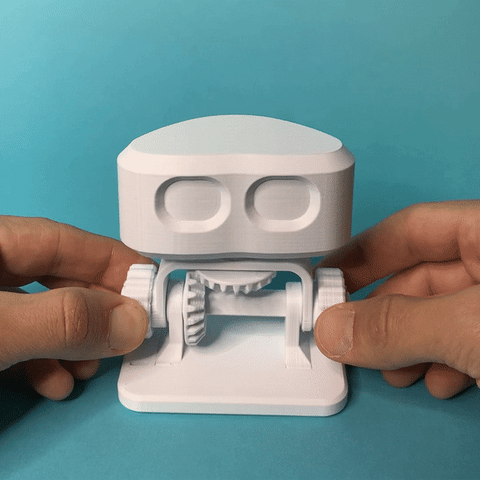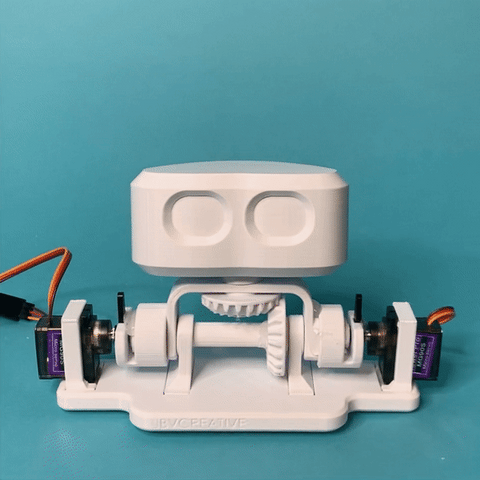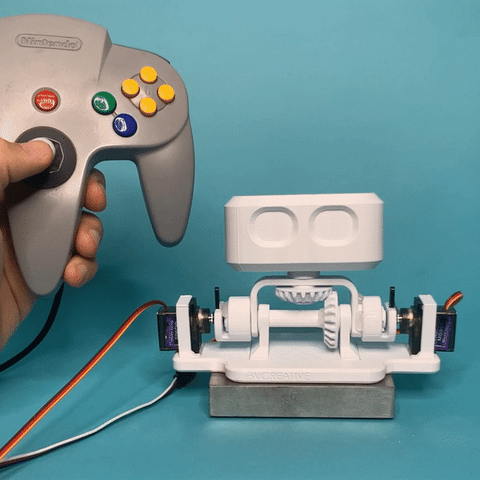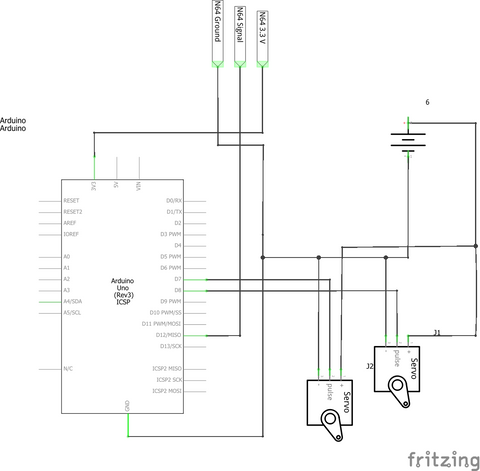# The Robot Head Project - 2 Degree of Freedom Gimbal

I originally saw the mechanism for this project on Youtube.  I wanted to create this in a fully 3d printable form because it's an interesting interactive mechanism.  I also thought it would make for a fun kinetic sculpture!

This video shows the engineering process behind RobBob the robot.

In the animation, 1 motor controls the rotation of the head through the yellow shaft and bevel gear.  The tilt of the head can be controlled by rotating both motors together.

In my mechanical 3d printed version I used knobs instead of motors:There is one design flaw, however, where if you only rotate the 'tilt' knob, the head will tilt and rotate. This is why you have to turn both knobs together to get the head to tilt without rotating.

After printing the first version, I thought it would be fun to swap the manual dials with some servo motors to automate the head.  Hooking up the motors to an Arduino board and writing a few lines of code allows it to be controlled digitally. Controlling things with code also allows you to address the tilt/rotation issue by adding only one additional line of code:

Actual_Rotation_Angle = Tilt_Angle + Desired_Rotation_Angle

This changes the angle of the rotation servo depending on the angle of the tilt servo. If you tilt without rotation, the servos move in sync.  If you then rotate, the rotation servo moves while keeping its centre position at the same angle as the tilt servo.The last thing required was a human interface which would allow the robot to be puppeteered.  At first I was thinking of using the Serial monitor to send the robot head commands.  This would've been a little clunky, so instead I opted for a joystick. I could have bought one like this, but before spending the money, I wanted to take a crack at using the joystick of an old N64 controller.  A quick google search revealed this library, which makes interfacing an Arduino with an N64 incredibly easy.This is the schematic:This is a fun kinetic sculpture, however there are practical uses for a pan/tilt mechanism like this one.  One practical application would be to mount a camera instead of the head.  This would allow you to move the camera around to see more of your environment.

Here is the code:

#include <Servo.h>
#include <N64Controller.h>

Servo tiltServo;
Servo rotationServo;

N64Controller player1(12); //Set up N64 Controller to pin 12

void setup() {
player1.begin(); // Initialisation
Serial.begin(9600);

tiltServo.write(90);
rotationServo.write(90);
tiltServo.attach(7);
rotationServo.attach(8);
}

void loop() {
delay(5);
//Get a reading from the controller and place it into variables
player1.update();
int x_value = int(player1.axis_x());
int y_value = int(player1.axis_y());

//This is a correction to allow for more head rotation while the
if (y_value > 30)
{
y_value = 30;
} else if (y_value < -30)
{
y_value = -30;
}

//Make the correction to solve the tilt/rotation problem
x_value = x_value + y_value;

//Map the joystick values to the motor angles
x_value = map(x_value,-70,70,180,0);
y_value = map(y_value,-70,70,0,170);

//Write the angles to the motors!
rotationServo.write(x_value);
tiltServo.write(y_value);

Serial.print("X value: ");Serial.println(x_value);
Serial.print("Y value: ");Serial.println(y_value);
}